# From force to field

### About fields – a central concept that is relevant not only for gravitational physics

#### An article by Markus Pössel

The description of forces acting at a distance – e.g., the electrostatic force, by which a particle with electric charge can influence other charged particles – usually involves at least two particles acting on each other, never just one. This isn’t without reason, because in general the strength of such a force between two particles A and B is proportional to the charge of particle A as well as to the charge of particle B. Therefore, without knowing the charges of both particles, the strength of the force cannot be determined at all.

Nevertheless, in many situations, it is meaningful and useful to describe the influence of charged particles without immediately bringing a concrete target object of this influence into play. The physical quantities suitable for this are called force fields or, somewhat more general, shorter and far more usual, fields.

## From sign to arrow

Let us consider the simplest case, the electric field around a charged metal sphere, which belongs to the electrostatic force. Figuratively, we can think of it as if at every point in space around the metal sphere, a small sign like the following would be floating: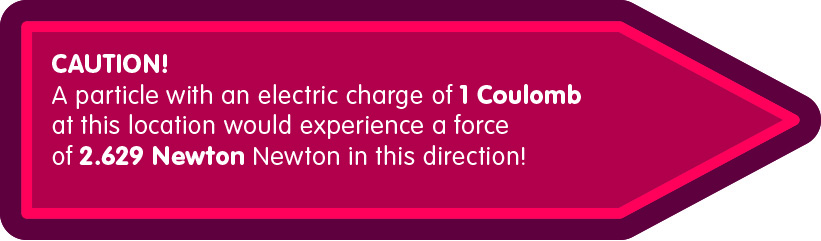If you want to find out the force on a particle which is at a certain place, you just have to read the imaginary sign at this place. It indicates the force exerted by our metal sphere on a reference particle with an electric charge of 1 Coulomb. If the particle we are concerned with has a larger or smaller electric charge than 1 Coulomb, the acting force will accordingly be larger or smaller. Specifically, if our particle has a charge of X coulombs, then the force acting on it is X times the force on the reference particle noted on the plate.

Another convention takes into account that positively and negatively charged particles feel opposite electrical forces in the same situation: where a positively charged particle is repelled, a negatively charged one is attracted. Our reference particle is positively charged by definition, and therefore the following applies: If the charge of the particle we consider (and thus the factor X) is negative, then a force acts on this particle exactly opposite to the direction of the arrow of the sign.

Of course, it is unnecessary to repeat the information which is the same on every sign – that the statement applies to a particle of the charge 1 Coulomb and that the quantity stated is a force. What really matters are only two pieces of information that must be known at each location: The strength and the direction of the force on the reference particle. No actual sign is needed for these two pieces of information, a simple arrow is sufficient, in the language of mathematicians a “vector”:

Here, the length of the arrow indicates the strength of the force experienced by the test particle located at the base point P, and its direction indicates the direction of action of the force. Here is an example: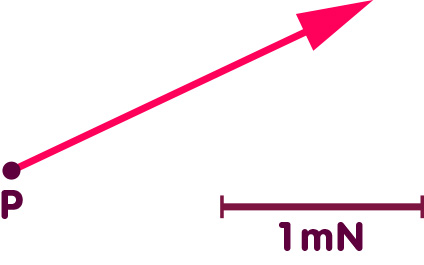Comparing the length of the arrow with the specified length scale that should apply to our arrows – a distance that should correspond to one thousandth of one Newton, or millinewton, mN, as the length of the arrow – shows that a force of 2 millinewtons acts on a reference particle at the drawn location P in the direction of the arrow.

## A crowd of arrows

Strictly speaking, one such field vector is defined for each point in space. The totality of all these arrows form the electric field. In a visual representation, of course, one will actually draw only very few of these vectors – just enough so that the individual vectors can be recognized, and that the totality of vectors gives a good overview of the properties of the field in question. Here are some representative arrows that give us an overview of the electric field around a charged metal sphere that carries a charge of 2 Coulombs and has a diameter of 16 centimeters: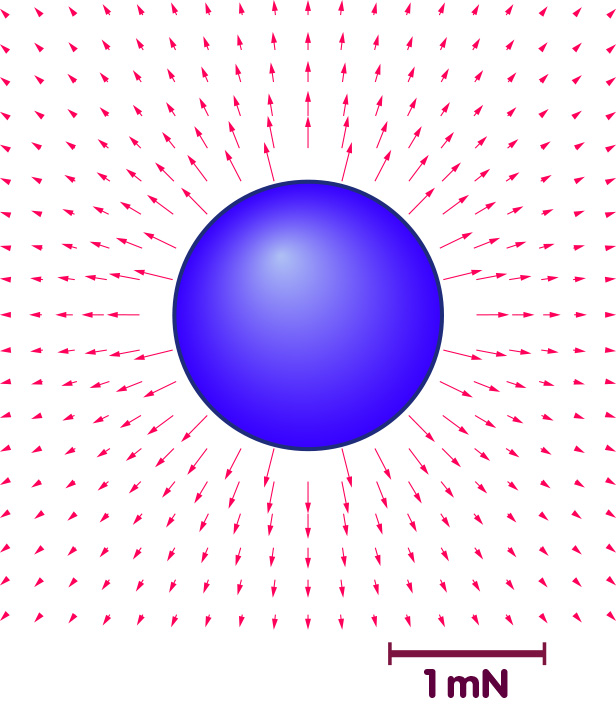Clearly indicated by the vector arrows: The force is directed directly away from the center of the sphere at each location. This is not unexpected: The positively charged sphere electrically repels our positive reference particle. The further we move away from the center of the sphere, the shorter the arrows become. This is no surprise either: the electrical force between two charges is smaller the further apart the charges are. By precisely measuring the lengths of the arrows, one can also read from the figure how the strength of the force depends on the distance: it is inversely quadratic, i.e. at double distance, the force is only a quarter as strong as before.

## The gravitational field

In analogy to the electric field, the field belonging to Newton’s gravitational force can be defined as well: Each arrow indicates the force acting on a reference particle with a mass of one kilogram. In one respect the situation is even simpler than with the electric force: There is only one kind of mass, and all masses attract each other. The arrows – the field vectors – therefore directly indicate the direction of the force in each case, without having to worry about positive and negative charges.

In the following figure selected field vectors around a spherical central mass like the earth are shown: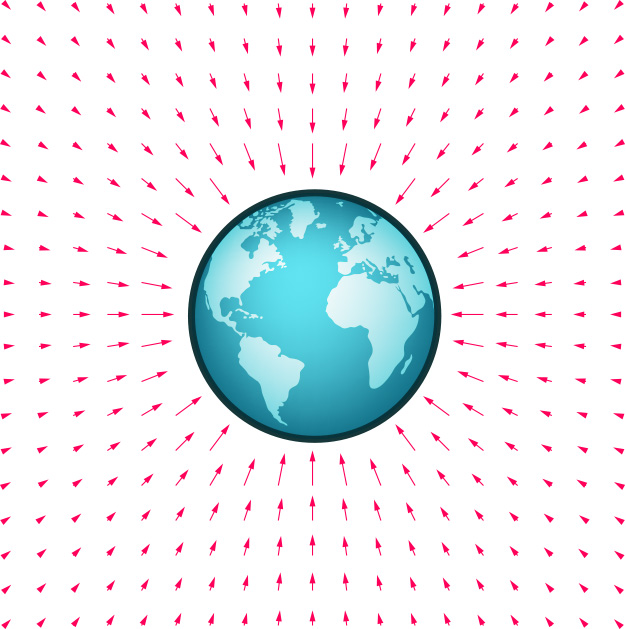All field vectors point directly to the center of the Earth – this is where the gravitational force of the globe is directed, acting on all particles in the vicinity of our planet. The strength of the gravitational force – the length of the arrows – decreases with the square of the distance.

## Magnetic fields

For the electric field and the Newtonian gravitational field, the field vectors are directly aligned with the force acting on the reference particle. For magnetic fields, the relationship is somewhat more indirect, but again, the following applies: the force exerted by a magnet on passing electrically charged particles is associated with a field that assigns a magnetic field vector to each point in space. As an example, the following figure shows the magnetic field vectors of a simple bar magnet with north and south poles: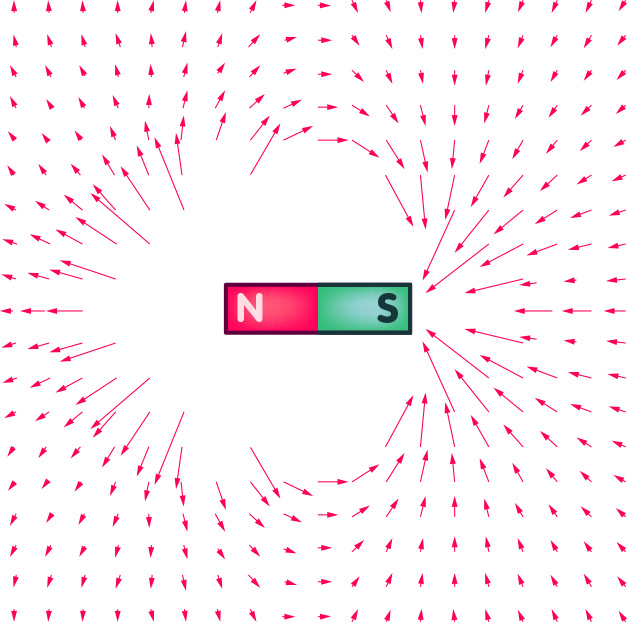Only vectors starting at a certain minimum distance from the magnet are shown. Vectors whose base points are further inside would be so long that they would intersect the outer vectors and make the figure unclear.

One meaning of the magnetic field vectors is easy to understand: If a small magnet is put into the existing magnetic field, then forces act trying to rotate the small magnet so that its orientation is exactly parallel to the magnetic field arrow (with the north pole in the direction of the arrow):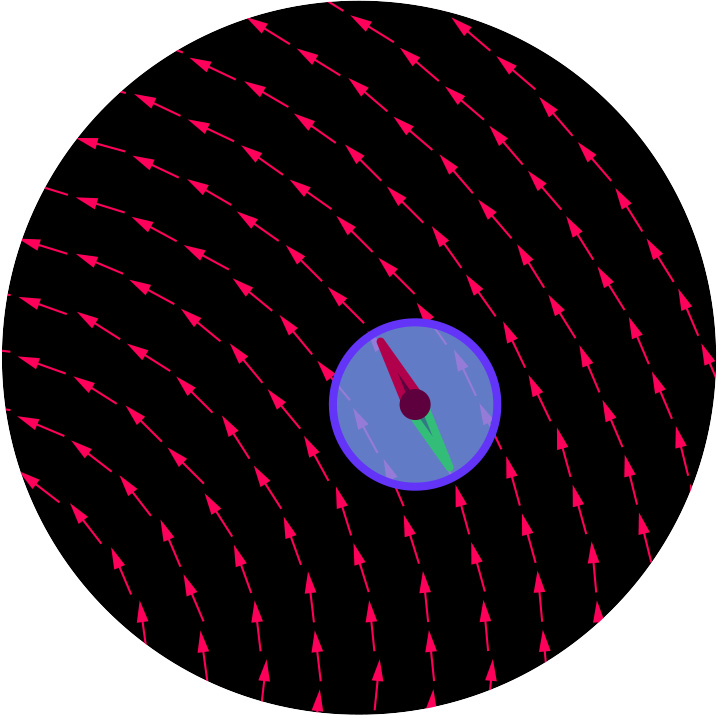This effect has a highly practical application: it ensures that a freely rotating compass needle points north.

More generally, magnetic fields are accompanied by a force on moving electric charges. However, it does not act directly in the direction of the field arrow, but somewhat differently: the speed of a particle can also be assigned an arrow – the length of the arrow indicates how fast the particle is moving, the direction of the arrow indicates in which direction it is flying. The direction of the deflecting force is derived from the so-called right-hand rule: spread the thumb, index finger and middle finger of your right hand so that two of the fingers are perpendicular to each other – the thumb extended, the index finger straight and the middle finger at right angles to the palm. Let’s assume that your thumb points in the direction of the magnetic field vector, and your middle finger points in the direction of the velocity of an electrically charged particle in this magnetic field – more precisely: in the direction of that part of the velocity which corresponds to a movement perpendicular to the magnetic field vector. Then the particle experiences a force in the direction of your index finger, as sketched in the following figure:The magnitude of the force is the product of the velocity, the strength of the magnetic field, and the electric charge of the particle.

For a more general direction of motion of the particle, one uses the just mentioned mnemonic by considering only that part of the particle speed that is perpendicular to the magnetic field, in order to get from the field to the force.

## The dance of fields and particles

After the previous descriptions it may seem as if force fields are only an auxiliary construction to describe distant forces between matter particles. In fact, however, there are good reasons to regard particles and fields as equally real. Let us take the electromagnetic field as an example: Just like particles, electric and magnetic fields also have an energy – a region of space in which the electric or magnetic field deviates from zero contains corresponding energy. In addition, electromagnetic fields do not only occur rigidly bound to electrically charged objects or magnets as in the picture examples shown above. Under suitable circumstances, field configurations occur which, following the basic equations of electromagnetism, propagate through space almost completely independently: These are electromagnetic waves such as light.

Therefore, the events in a region of the universe filled with electrically charged particles result, from today’s point of view, from the interaction of fields and particles: Where the charged particles are located and how they move determines the properties of the electric and magnetic fields that fill space. The electric and magnetic fields, in turn, affect particle motion: As discussed in the definition of electric and magnetic fields above, electric fields result in a force on charged particles, magnetic fields result in a force on moving charged particles. The changed positions and the changed motion of the particles lead to corresponding changes of the fields, which in turn influence the particle motion thereupon somewhat differently, and so on and on in an endless dance of fields and particles.

Whoever wishes to describe the evolution of such a particle-field ensemble must describe this dance, in which particles and fields mutually influence each other.

To put it more mathematically, the following applies: The electric and magnetic fields that result for a given charge configuration are determined by the so-called field equations. How the particle motion is influenced by the fields is governed by the so-called equations of motion. If you want to describe the evolution of a particle-field ensemble, you have to solve the coupled system of equations consisting of both field equations and the equations of motion.

## Not only for vectors

In the above examples for fields, each point in space was associated with a field vector – a quantity with direction and length. More precisely, even each point in space at each instant can be assigned a field vector, since fields can change over time, as explained in the last section.

In general, we are dealing with a field whenever a value of a certain physical quantity is assigned to every point in space at every point in time. This need not be a vector, but may well be other types of quantities. In certain situations, such as describing a star, it may be useful to assign a temperature value to each region of space, indicating the temperature of the stellar gas it contains. This is not a vector, but a simple numerical value.

On the other hand, there are more complicated possibilities: In general relativity, a quantity defined at each point, called “metric field” or, in short, “metric”, describes the geometry of the space region in question at the given time. This quantity thus contains all the information needed to describe how the geometry of the corresponding spacetime is distorted. In general relativity, the distortion is directly related to gravity, and in this sense the metric is something like the gravitational field of the theory.

## Further Information

This section introduces the notion of field, which is fundamental in both special and general relativity (and not only there). Basic information on the fundamentals of both theories is provided in the chapters Special Relativity and General Relativity in Elementary Einstein.

###### Colophon
Markus Pössel

is the managing scientist at Haus der Astronomie, the Center for Astronomy Education and Outreach in Heidelberg, and senior outreach scientist at the Max Planck Institute for Astronomy. He initiated Einstein Online.